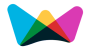#Square and Cube Calculator

Calculates the diagonal,perimeter,area of a square and diagonal,surface area,volume of a cube.

square units

square units
cubic units## Diagonal, Area of a square, Surface area, Volume of a cube Formulas

Diagonal of a square =  2  × Side Length

Area of a square = Side Length2

Perimeter of a square = 4 × Side Length

Surface area of a cube = 6 × Side Length 2

Volume of a cube = Side Length3

Diagonal of a cube =  3  × Side Length

Definition :

1. What is perimeter?

The length around a shape.

2. What is area?

The number of squares inside a shape.

3. What is volume?

The number of cubes that fit inside a shape.

4. What is surface area?

A. The area of all the surfaces of a 3-D shape.

###More Calculators ►

Online math number calculation, formulas